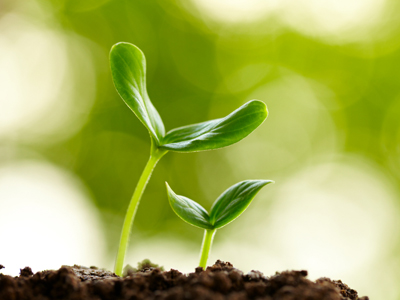If you were carrying out an investigation into the effect of light on seed germination, you would change the amount of light to which the seeds are exposed.

# Investigations 02

Dive into the world of experiments with this second KS2 Science quiz on investigations! Learn about variables and equipment used to ensure fair results.

Investigations are exciting and involve experiments, predictions, methods, equipment, and variables. Planning investigations in science classes is lots of fun and helps you develop logical and methodical skills. It's also how scientists discover new facts about the world. Explore questions about variables, equipment, and fair tests in this second Investigations quiz!

1.
If you were carrying out an investigation into the effect of light on seed germination, how would you measure the dependent variable?
By how tall the shoots grow in a certain number of days
By the number of days it takes the seeds to germinate
By the number of seeds which germinate after a certain number of days
Either the number of days it takes or by the number of seeds which germinate
The amount of light might also affect how much the shoots grow, but we are only interested in germination in this investigation
2.
If you were testing the effect of water resistance on three different plasticine shapes, which variable would you be changing?
The mass of the plasticine shape
The shape of the plasticine
The amount of water in the beaker
All of the above
You would have to make sure the mass (weight) of each of the three shapes was exactly the same
3.
If you were carrying out an investigation into the effect of light on seed germination, what would be the dependent variable?
Whether the seeds germinate
How many seeds you plant
How tall the plants grow
How much light you give the seeds
The dependent variable is the thing we are measuring - in this case, seed germination
4.
If you were carrying out an investigation into the effect of light on seed germination, which variable would you change?
The amount of water you give the seeds
The temperature of the places where you've left the seeds
The amount of light to which the seeds are exposed
The types of seeds which will be germinating
Only the amount of light should be changed - all other variables would be kept the same
5.
What unit of measurement would you use to record the amount of time it takes different shapes to reach the bottom of a beaker of water?
Centimetres
Degrees Celsius
Seconds
Millilitres
Because you would be measuring the amount of time it takes, you would need to measure in seconds
6.
You are testing materials to see whether they conduct electricity. You have built a circuit of a battery and two crocodile leads which you will attach to the material. What is missing?
A switch
A light bulb, buzzer or motor
A parallel circuit
Nothing is missing
Without a light bulb, buzzer, or motor, you will not be able to see whether electricity can pass through the material you are testing - if electricity can pass through, the buzzer will buzz, the motor will run or the bulb will light up
7.
If you were testing materials to see whether they conduct electricity using a circuit and bulbs, what variable would you be changing?
The strength of the battery
The number of bulbs
The material
The time it takes the electricity to complete the circuit
The materials are what are being tested so they are the only thing that should be changed
8.
If you investigated the effect of water resistance on different shapes moulded from plasticine by dropping them in a beaker of water and timing how long it takes them to reach the bottom, what would happen if you changed the amount of water, the shape of the plasticine and the mass of the different shapes?
Your results would all be the same
Your investigation would take too much time
The investigation would not be a fair test
You wouldn't have enough time to display your results
As it's the shapes we are investigating, only the shapes and nothing else must change
9.
If you were testing materials to see whether they conduct electricity using a circuit and bulbs, what would be the dependent variable?
The length of the crocodile leads
How long the investigation takes
The strength of the battery
Whether electricity passes through the material
The dependent variable is the variable you measure - here it would be measured by whether the bulb works
10.
You would like to investigate the effect of water resistance on different shapes moulded from plasticine. You are dropping the shapes in a beaker of water. What is the dependent variable?
The amount of time it takes the shape to reach the bottom of the beaker
The amount of water in the beaker
The mass of the plasticine shape
The colour of the plasticine
We are measuring water resistance which can be seen from the amount of time it takes an object to sink - a longer time means more water resistance and a shorter time means less